## 11/29/2011

### (TIP) Matlab Plot Option

colormap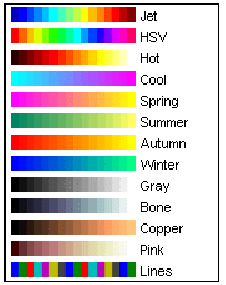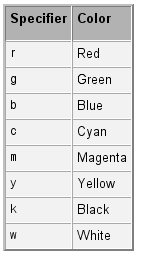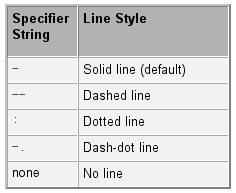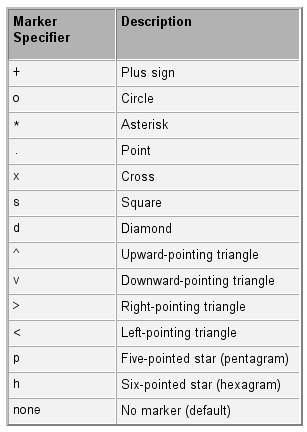Below code is example of matlab help document.
x = -pi:pi/10:pi;
y = tan(sin(x)) - sin(tan(x));
plot(x,y,'--rs','LineWidth',2,...
'MarkerEdgeColor','k',...
'MarkerFaceColor','g',...
'MarkerSize',10)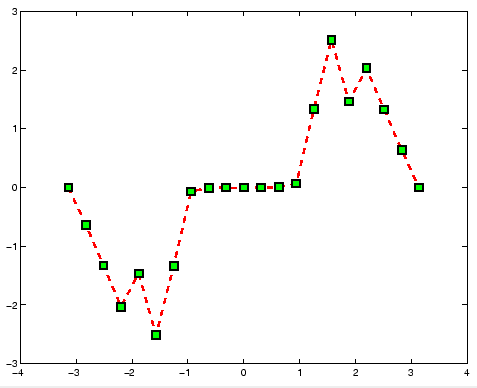You can describe axes on your image.
figure(1);
iptsetpref('ImshowAxesVisible','on');
imshow(data2); %'colormap',bone(0)
set(gca, 'XTickLabel', {'a', 'b', 'c', 'd', 'e', 'f'} );
set(gca, 'YTickLabel', {100:100:700} );
xlabel('Longitude');ylabel('Latitude');

## 11/27/2011

### (TIP) Making a file name appending number in Matlab

It is simple and easy but I can not remember the code well when I need this code.
So I leave this code in my blog.
And I hope the code is also useful to all visitor.
Thank you.

endIndex = 10;
for j=1:endIndex
Index = num2str(j);
end

->
This source code can load below file names sequentially.
1saveFile.txt
2saveFile.txt
3saveFile.txt
4saveFile.txt
5saveFile.txt
6saveFile.txt
7saveFile.txt
...

## 11/25/2011

### Screen capture and save program/ C++ source code

Created Date : 2011.11
Language :C++
Tool : Visual Studio 2008
Library & Utilized : CImage
Reference : Internet
etc. : -

I made this program to help my friend.
This progrma is screen capture program.
At first, we do whole screen capture and set region of screen to capture.
Then start capture and image saving in real-time.
The image file is saved in your program directory.
The file name of the saved image is like that "Time.jpg(EX: 11223242.jpg, 11223243.jpg...)"

The program is made based on below capture program.

```#include
void Capture()
{

int nx =0, ny = 0;
CImage capImage;
CWnd *pDesktopWnd = GetDesktopWindow();
HDC hDC = NULL;
if(!pDesktopWnd)
return;
CWindowDC DeskTopDC(pDesktopWnd);
nx = GetSystemMetrics(SM_CXSCREEN);
ny = GetSystemMetrics(SM_CYSCREEN);
if(!capImage.Create(nx, ny, 32))
return;
hDC = capImage.GetDC();
BitBlt(hDC, 0,0, nx, ny, DeskTopDC.m_hDC, 0, 0, SRCCOPY);
capImage.Save(_T("test.jpg"), Gdiplus::ImageFormatJPEG);
capImage.ReleaseDC();

}
```

I am hard to make file name of capture image because the progrma requires UNICODE type character.

So I solved this problem as below code.

```    int T = time(NULL);
TCHAR str;
wsprintf(str,_T("%d.jpg"),T);
ImageTemp.Save(str, Gdiplus::ImageFormatJPEG);
ImageTemp.ReleaseDC();
```

source code

## 11/16/2011

### Thread Test in the C++ console mode.

The goal of this source code is how to send large data to the thread and how to wait until all thread finish their job.

I use struct to send the large data.
and I use the flags for waiting the time of the all thread finish.

<source code>

```//////////////////////////////////////////////////////////////////////////
// Made by J.H.KIM, 2011 / feelmare@daum.net, feelmare@gmail.com        //
// blog : http://feelmare.blogspot.com                                  //
// My Lab : VISLAB(http://me.pusan.ac.kr)                               //
//////////////////////////////////////////////////////////////////////////

#include <stdio.h>
#include <process.h>
#include <windows.h>

struct InputData
{
int * data;
int width;
int height;
int OriginW;
int reserved;
int flag;
};

void main()
{

//Data Allocation and Setting
int W = 100;
int H = 100;
int * buffer;
buffer =new int[W*H];

for(int i=0; i<W*H; ++i)
{
buffer[i] = i;
}

int sx,sy,w,h;

InputData th1_input;
sx = 0; sy = 0; w = 80; h = 90; //(80x90) image processing
th1_input.width = w;
th1_input.height = h;
th1_input.OriginW = W;
th1_input.data = &buffer[sy*W+sx];
th1_input.reserved = 1;
th1_input.flag = TRUE;

InputData th2_input;
sx = 10; sy = 10; w = 50; h = 50; //(50x50) image processing
th2_input.width = w;
th2_input.height = h;
th2_input.OriginW = W;
th2_input.data = &buffer[sy*W+sx];
th2_input.reserved = 2;
th2_input.flag = TRUE;

InputData th3_input;
sx = 10; sy = 10; w = 20; h = 20; //(10x10) image processing
th3_input.width = w;
th3_input.height = h;
th3_input.OriginW = W;
th3_input.data = &buffer[sy*W+sx];
th3_input.reserved = 3;
th3_input.flag = TRUE;

//wait until all thread is finished.
while( th1_input.flag || th2_input.flag || th3_input.flag)
{
Sleep(100);
}

//Data buffer release
delete[] buffer;

}

//copy struct data to variable
int w = ((InputData*)pParam)->width;
int h = ((InputData*)pParam)->height;
int ow = ((InputData*)pParam)->OriginW;

//Processing Sum(v^2.)
int v;
double sum=0;
for(int j=0; j<h; ++j)
{
for(int i=0; i<w; ++i)
{
v = ((InputData*)pParam)->data[j*ow+i];
sum += (v*v);
}
}

printf("%d thread value = %lf\n", ((InputData*)pParam)->reserved , sum);

((InputData*)pParam)->flag = FALSE;
}

```

Please leave comment if the source code has problem.
Thank you~

## 11/15/2011

### Web Cam Capture source code using OpenCV

This source code is very simple. But the code will be help to somebody who start to study the opencv.
Thank you~.

```    //image class
IplImage* image = 0;
//camera point
CvCapture* capture = cvCaptureFromCAM(0);
//make window
cvNamedWindow( "camera");

//image class for processing
IplImage* image2=0;
//make window
cvNamedWindow( "camera2");

unsigned char R,G,B;
while(1) {
//capture a frame form cam
cvGrabFrame( capture );
//copy to image class
image = cvRetrieveFrame( capture );

//create image at one time in the roof
if(image2 == 0)
{
image2  = cvCreateImage(cvSize(image->width, image->height), image->depth, image->nChannels);
}

//simple image processing
for(int i=0; i<image->height; ++i)
{
for(int j=0; j<image->width; ++j)
{
//to get R,G,B value
B = (unsigned char)image->imageData[i*image->widthStep+j*3+0];
G = (unsigned char)image->imageData[i*image->widthStep+j*3+1];
R = (unsigned char)image->imageData[i*image->widthStep+j*3+2];

//image color converting
B = 255-B;
G = 255-G;
R = 255-R;

//copy R,G,B value to image2
image2->imageData[i*image2->widthStep+j*3+0] = B;
image2->imageData[i*image2->widthStep+j*3+1] = G;
image2->imageData[i*image2->widthStep+j*3+2] = R;
}
}

//show window
cvShowImage( "camera", image );
cvShowImage( "camera2", image2 );

//break
if( cvWaitKey(10) >= 0 )
break;
}

//release imge
cvReleaseImage(&image2);
//release capture point
cvReleaseCapture( &capture );
//close the window
cvDestroyWindow( "camera" );
cvDestroyWindow( "camera2" );
```

## 11/11/2011

### Project - Embeded camera R&D for Real-Time fire detection surveillance in tunnel environment

Embeded camera R&D for Real-Time fire detection surveillance in tunnel environment
- 2008.07.01~2009.06.30
- High Computing Power Embeded Camera R&D, Fire & Smoke Detection(My Job)

The goal of the project is to develop embeded camera for detect fire and smoke.
My job was an algorithm R&D for detection fire & smoke and the s/w programing.
The fire detection algorithm uses the HMM algorithm.
The features of the fire's sequence is learned in the off-line. And then the learned Markov model is used in the oline using viterbi algorithm.

Below Movies are the result of the project.

*** Demo 1, Fire detection Simple situation :

*** Demo 2, Fire detection in the road, There is similar light of the car with fire color:

***Demo 3, Fire detection test in the real fire situation :

***Demo 4, Embeded Camera Test. The camera detects the fire and then zoom in the region of the fire.

***Demo 5, Smoke detection test.

## 11/08/2011

### 8 point algorithm (Matlab source code) / The method to get the Fundamental Matrix and the Essential matrix

Created Date : 2011.8
Language : Matlab
Tool : Matlab 2010
Library & Utilized : -
Reference : Multiple View Geometry (Hartly and Zisserman)
etc. : Intrinsic Parameter, 2 adjacent images, matching points

This code is 8 point algorithm.
If we know over 8 corresponding points between two images, we can know Rotation and Translation of camera movement using 8 point algorithm.
The 8 point algorithm is well known in the vision major field.
The algorihtm is introduced at the Multiple View Geometry Book and many websites.

Have you ever listened Fundamental matrix song? The song is very cheerful. ^^

My code is very simple. so I believe my code will be useful to you.
I will upload RANSAC version later.
Thank you.

github url :https://github.com/MareArts/8point-algorithm
(I used 'getCorrectCameraMatrix' function of Isaac Esteban author.)

main M code..
```%//////////////////////////////////////////////////////////////////////////
%// Made by J.H.KIM, 2011 / feelmare@daum.net, feelmare@gmail.com        //
%// blog : http://feelmare.blogspot.com                                  //
%// Eight-Point Algorithm
%//////////////////////////////////////////////////////////////////////////

clc; clear all; close all;

% Corresponding points between two images
% sample #1 I11.jpg, I22.jpg
%{
m1 = I11; m2 = I22;
%}

%sample #2 I1.jpg, I2.jpg
m1 = I1; m2 = I2;

s = length(m1);
m1=[m1(:,1) m1(:,2) ones(s,1)];
m2=[m2(:,1) m2(:,2) ones(s,1)];
Width = 800; %image width
Height = 600; %image height

% Intrinsic Matrix
K = intrinsic_matrix;

% The matrix for normalization(Centroid)
N=[2/Width 0 -1;
0 2/Height -1;
0   0   1];

%%
% Data Centroid
x1=N*m1'; x2=N*m2';
x1=[x1(1,:)' x1(2,:)'];
x2=[x2(1,:)' x2(2,:)'];

% Af=0
A=[x1(:,1).*x2(:,1) x1(:,2).*x2(:,1) x2(:,1) x1(:,1).*x2(:,2) x1(:,2).*x2(:,2) x2(:,2) x1(:,1) x1(:,2), ones(s,1)];

% Get F matrix
[U D V] = svd(A);
F=reshape(V(:,9), 3, 3)';
% make rank 2
[U D V] = svd(F);
F=U*diag([D(1,1) D(2,2) 0])*V';

% Denormalize
F = N'*F*N;
%Verification
%L1=F*m1'; m2(1,:)*L1(:,1); m2(2,:)*L1(:,2); m2(3,:)*L1(:,3);

%%
%Get E
E=K'*F*K;
% Multiple View Geometry 259page
%Get 4 Possible P matrix
P4 = get4possibleP(E);
%Get Correct P matrix
inX = [m1(1,:)' m2(1,:)'];
P1 = [eye(3) zeros(3,1)];
P2 = getCorrectCameraMatrix(P4, K, K, inX)

%%
%Get 3D Data using Direct Linear Transform(Linear Triangular method)
Xw = Triangulation(m1',K*P1, m2',K*P2);
xx=Xw(1,:);
yy=Xw(2,:);
zz=Xw(3,:);

figure(1);
plot3(xx, yy, zz, 'r+');

%{
%This code is also run well instead of Triangulation Function.
nm1=inv(K)*m1';
nm2=inv(K)*m2';
% Direct Linear Transform
for i=1:s
A=[P1(3,:).*nm1(1,i) - P1(1,:);
P1(3,:).*nm1(2,i) - P1(2,:);
P2(3,:).*nm2(1,i) - P2(1,:);
P2(3,:).*nm2(2,i) - P2(2,:)];

A(1,:) = A(1,:)./norm(A(1,:));
A(2,:) = A(2,:)./norm(A(2,:));
A(3,:) = A(3,:)./norm(A(3,:));
A(4,:) = A(4,:)./norm(A(4,:));

[U D V] = svd(A);
X(:,i) = V(:,4)./V(4,4);
end
%}

```

## 11/07/2011

### Rotation Matrix Converting Matlab Source (Euler Angle, Rotation Matrix, Quanternion)

There are many expression to show the rotation value.
(Ex. : Euler, Matrix, Quaternion.. )

This code is the test source to convert each other.
Euler -> Matrix -> Quanternion -> Matrix -> Euler
We can show the first Euler value is same with the last Euler value.

The source code is like below:
--------------------------------------------------------------

% Rotation vector of x,y,z axis.
Rv = [13 20 50];

% 3x3 matrix of R vector (Results of the Rm1 and Rm2 is similar.)
Rm1 = rodrigues(Rv*pi/180)
Rm2 = mRotMat(Rv)

% Quntenion vector of R matrix
Rq1 = matrix2quaternion(Rm1)
Rq2 = matrix2quaternion(Rm2)

% R matrix of Q vector
Rm1_1 = quaternion2matrix(Rq1)
Rm2_2 = quaternion2matrix(Rq1)

% R vector of R matrix
Rv_1 = rodrigues(Rm1_1(1:3,1:3)) * 180/pi
Rv_2 = rodrigues(Rm2_2(1:3,1:3)) * 180/pi

-----------------------------------------------------------------------
<Source Code>

The copyright of "rodrigues' and 'quaternion' functions is reserved by Peter Kovesi.

I wish this source code is useful to you.
Thank you.

## 11/04/2011

### A* path planning Algorithm / C++ source code

Created Date : 2010.8
Language : C/C++
Tool : Microsoft Visual C++ 6.0
Library & Utilized : STL
Reference : A* internet reference
etc. : map(.txt file)

It is A* algorithm. A* is known as good path plan algorithm in the Game and other fields.
I made the A* algorithm program. I have refered a lot of reference in the internet. You also can get materials easily in the internet.

I made the algorithm to class type. So we can use the A* Class like this;

CAstar Astar;
Astar.MapCopy(xSize, ySize, &map);
Astar.SetSEpoint(sX, sY, eX, eY);
vector< pair<int, int > > path = Astar.FindPath();

map variable type is 'int ** map'.
sX, Sy is start coordinate. eX, ey is  end coordinate.
The path coordinate is saved in the path variable(vector type).